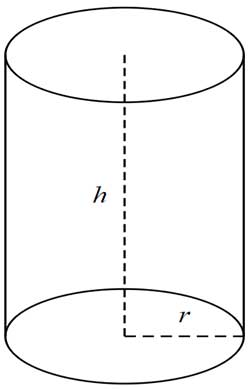# Cylinder Volume and Surface Area Calculator

Calculator

Do you need to calculate the volume and/or the surface area of a cylinder? Thanks to our free Cylinder Volume and Surface Area Calculator you can calculate it online, in no time.

Q&A

## 1. How to calculate the cylinder volume?To calculate the volume of a circular cylinder you need the radius of the base of the cylinder (r) and the height of the periphery of the cylinder (h). If you only have the diameter of the cylinder base than you can get the length of the radius by dividing the diameter by 2. Use the following formula to calculate the cylinder volume, which is the same as the one used by the Cylinder Volume Calculator:

Cylinder Volume calculation formula = π*r2*h

The π (pi) used in the formula is the quotient of the circumference and the diameter of the circle, which is a constant and is equal to 10 decimal places: 3.1415926535

### 2. How to calculate the cylinder surface area?

You need the radius of the base of the cylinder (r) and the height of the periphery of the cylinder (h) to calculate the surface area of the circular cylinder. (The radius of the base diameter is obtained by dividing the diameter by two). To calculate the surface of a cylinder, use the following formula, which is the same as the one used by the Calculator of Surface Area

Formula for calculating the Surface Area of the Cylinder = 2*π*r*h+2*π*r2 or you can use this simplified formula= 2*π*r(r+h)

### 3. How do the Cylinder Volume and Surface Calculator calculate?

The calculator uses the formulas described in the previous sections to calculate the cylinder volume and surface area. When you enter data into the calculator, you can also specify the unit of measurement of the data.